## Summary

Electroweak Calibration of the Higgs Characterization Model (my CERN research project). Particle physicists use a theory called the Standard Model to predict physics in the Large Hadron Collider. I used two Monte Carlo physics simulators to see what happens to numerical models of Higgs physics when we leave out the electroweak force.

### Background

How does the Higgs boson discovered at the Large Hadron Collider in 2012 resemble the scalar particle predicted by the Standard Model? We used a model-independent Effective Field Theory (EFT) approach to search for interesting deviations from Standard Model physics.

We focused our efforts on the HZ Higgs production mode known as Higgs radiation. When two protons collide in the Large Hadron Collider, energized gluons emit a high-energy Z boson which then radiates a Higgs boson before decaying into two muons.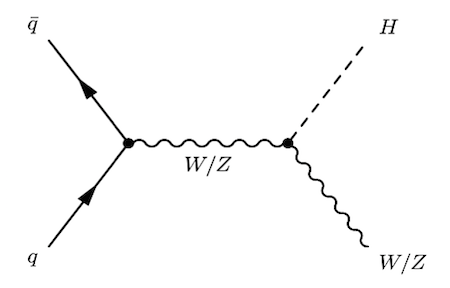We wanted to learn how electroweak corrections affected EFT estimates. More concretely, we sought to calibrate a popular EFT model, the Higgs Characterization (HC) Model, for electroweak contributions using simulated Higgs observabless.

### How it works

The Higgs Characterization Model:

• Uses Effective Field Theory
• Assumes only Standard Model fields and symmetries
• Can be used to search for new physics above the electroweak scale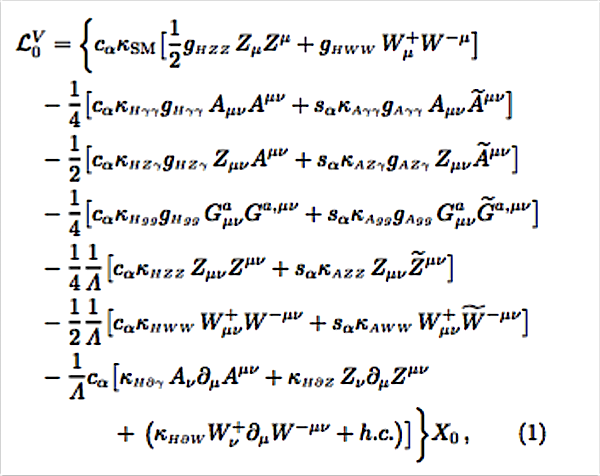The Higgs Characterization Lagrangian (FeynRules)

In this study, we ignored CP-odd terms (concretely, we set $$c_{\alpha}=1$$ and $$s_{\alpha}=0$$). We added $$\pm \epsilon$$ to scale factors $$\kappa_{H \gamma \gamma}$$, $$\kappa_{H Z \gamma}$$, $$\kappa_{H Z Z}$$, and $$\kappa_{H \partial W}$$ (where $$\epsilon$$ was a small constant).

First we used HAWK to calculate SM Higgs observables to next-to-leading-order (NLO) accuracy with quantum chromodynamic (QCD) and electroweak (EWK) contributions. The drawback of HAWK is that it only works in the SM domain; it cannot calculate BSM physics.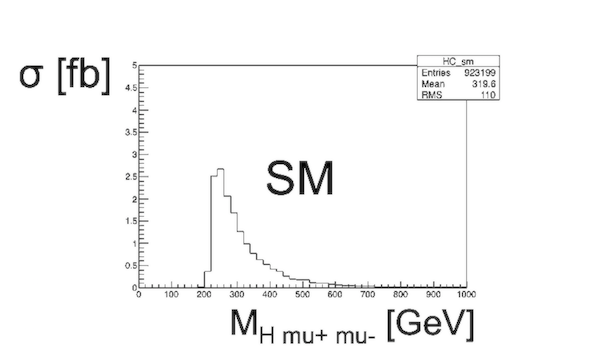Histogram of an observable of Higgs radiation, $$M_{Z \mu \mu}$$, calculated to next-to-leading order accuracy for both QCD and EWK

Next, we evaluated SM and eight BSM variants of the process to NLO accuracy just in QCD using the Higgs Characterization Model in MadGraph.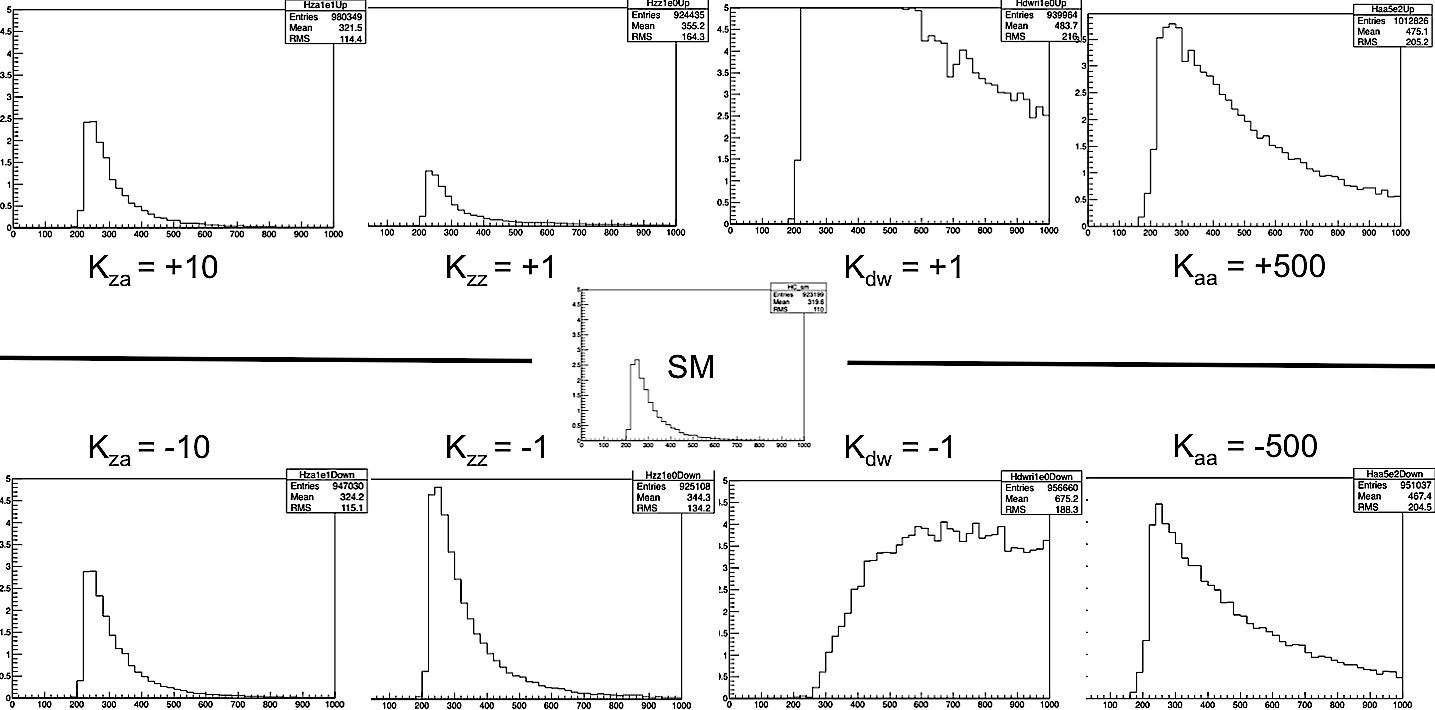BSM deformations (left to right) in $$\kappa_{H Z \gamma}$$, $$\kappa_{H Z Z}$$, $$\kappa_{H \partial W}$$, and $$\kappa_{H \gamma \gamma}$$

To compare the two, we used the Higgs Combine library to fit the Higgs Characterization Model results to their corresponding HAWK results. By treating the HAWK model as ‘observed data’ we were able to understand how and when electroweak forces resemble BSM couplings in the Higgs Characterization Model.

As luminosity increases, relative uncertainty in Large Hadron Collider data decreases. We increased the luminosity of our simulated data until the electroweak corrections became significant enough to resemble BSM physics. Our results enabled us to estimate the luminosities at which CERN will need to use electroweak corrections when exploring BSM physics.

### Discussion

Our preliminary results indicate that electroweak corrections are not significant until luminosities on the order of 3,000 fb$$^{-1}$$. The Large Hadron Collider will be operating at ~100 fb$$^{-1}$$ in the next year; we probably will not achieve 3,000 in the next 10 years.

I gave a formal presentation on this project on 10 December 2015. The recording and slides are on the Michigan CERN Research Abroad Program indico page.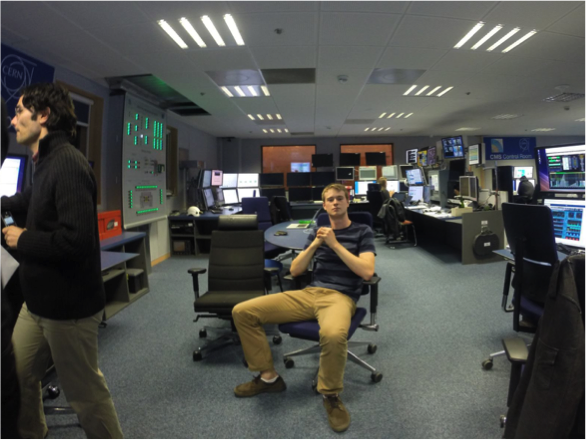The CMS control room